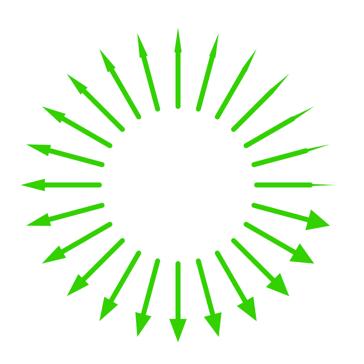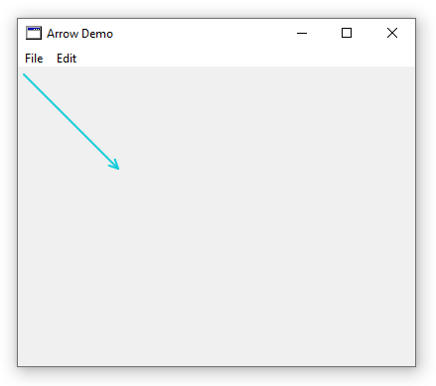#725 views

1. 6 months ago

### changwon l

17 Sep 2019 Pre-Release Testers

Seems to be quite easy question but I have no idea.

I want to draw a line with an arrow head. However, I couldn't find any method except below one.
g.Drawline

Can you let me know how to do it?

See post in context

The routine that I posted above doesn't account for pen size. For a one or two point pen size it won't be noticeable, but for a large pen size the arrowheads won't look good. Rather than leave things half done, I revised the routine to compensate for pen size, and while I was at it, I decided to include several arrowhead styles. A new property mArrowType has a range of 0..3 and the corresponding styles are:
0 - Open style (like the one given by Eugene Dakin)1 - Closed triangle2 - Filled triangle3 - Filled polygon (sharp point arrowhead)Revised code:

```Public Sub DrawArrow(extends g As Graphics, x1 As Double, y1 As Double, x2 As Double, y2 As Double)
'Draw a line with arrowhead at the end
'Calling parameters are the same as for DrawLine
'Arrowhead parameters are determined by properties arrowLength and arrowAngle
'
'*** Revised to compensate for large pen sizes
'
'Get angle of line
dim aLine As Double = ATan2(y2-y1,x2-x1)
dim ahLineA As Double = aLine-mArrowAngle*deg2halfrad
dim ahLineB As Double = aLine+arrowAngle*deg2halfrad
'calculate x and y coordinates of the arrowhead points
dim ahLineAx As Double = x2-mArrowLength*cos(ahLineA)
dim ahLineBx As Double = x2-mArrowLength*cos(ahLineB)
dim ahLineAy As Double = y2-mArrowLength*sin(ahLineA)
dim ahLineBy As Double = y2-mArrowLength*sin(ahLineB)
dim dPH as Double = g.PenHeight/2
dim dPW as Double = g.PenWidth/2
dim poly() As Double =Array(0,x2+dPW,y2+dPH,ahLineAx+dPW,ahLineAy+dPH,ahLineBx+dPW,ahLineBy+dPH)

if mArrowType=0 then
'Draw the principal line
g.DrawLine(x1,y1,x2,y2)
Else
'Draw the principal line (only to the base of the arrowhead)
g.DrawLine(x1,y1,(ahLineAx+ahLineBx)/2,(ahLineAy+ahLineBy)/2)
end if
if mArrowType<3 then
'Draw two line segments for arrowhead
g.DrawLine(ahLineAx,ahLineAy,x2,y2)
g.DrawLine(ahLineBx,ahLineBy,x2,y2)
end if
if mArrowType=1 or mArrowType=2 then
g.DrawLine(ahLineAx,ahLineAy,ahLineBx,ahLineBy)
end if
if mArrowType=2 or mArrowType=3 then
g.FillPolygon(poly)
end if
End Sub```

Project File

2. ### Dave S

17 Sep 2019 San Diego, California USA

there is no "built-in" draw an arrow routine.

use 3 drawline commands. and a little geometry

3. ### Scott C

I've been using the g.FillPolygon function to draw some simple triangles as part of some custom controls I've done on a Canvas.

I'm sure with a little patience and geometry, as Dave said, you could create a `DrawArrow` method that takes a few parameters, like `g As Graphics, x1 As Int32, y1 As Int32, x2 As Int32, y2 As Int32`, where `g` is a reference to your `Graphics` object in your `Paint` event, `x1` & `y1` is the "from" point of your arrow and `x2` & `y2` is the "to" point.

Making a separate `DrawArrow()` method could help with re-usability, if you need more than one arrow.

I could try to help with the geometry, but I'm afraid my head might explode in the process. I'm much more of a business logic guy than a graphics person.

I hope that helps.

4. ### Eugene D

17 Sep 2019 Pre-Release Testers, Xojo Pro Canada scispec.ca

Here is a method that draws a simple arrow with two lines for an arrow tip:

```Public Sub DrawArrow(g as Graphics, FromX as double, FromY as double, ToX as double, ToY as Double, AThickness as Integer, AColour as Color)
Dim HeadLength as Double = 10
Dim Pi as Double = 3.1415926

//Set the arrow properties
Dim Angle as Double = ATan2(ToY-FromY,ToX-FromX)
g.ForeColor = AColour
g.PenHeight = AThickness
g.PenWidth = AThickness
g.DrawLine(FromX, FromY,ToX, ToY)

End Sub```

Here is the line of code that is added to the Canvas Paint event:

```Sub Paint(g As Graphics, areas() As REALbasic.Rect) Handles Paint
//Draw an arrow
//g is graphics, FromX and FromY are the starting coordinates of the arrow
//ToX and ToY are the arrow end points
//AThickness is the arrow thickness
//AColour is the arrow colour
DrawArrow(g,5, 5, 100, 100, 2, &c11CCDD)
End Sub```

...and a screen grab of what the code makes....5. ### Robert W

Edited 6 months ago

Here's another version that uses a filled polygon for the arrowhead.

```module EnhancedGraphics

Public Sub DrawArrow(extends g As Graphics, x1 As Double, y1 As Double, x2 As Double, y2 As Double)
'Draw a line with arrowhead at the end
'Calling parameters are the same as for DrawLine
'Arrowhead parameters are determined by properties arrowLength and arrowAngle
'Get angle of line
dim aLine As Double = ATan2(y2-y1,x2-x1)
dim ahLineA As Double = aLine-mArrowAngle*deg2halfrad
dim ahLineB As Double = aLine+arrowAngle*deg2halfrad
'calculate x and y coordinates of the arrowhead points
dim ahLineAx As Double = x2-mArrowLength*cos(ahLineA)
dim ahLineBx As Double = x2-mArrowLength*cos(ahLineB)
dim ahLineAy As Double = y2-mArrowLength*sin(ahLineA)
dim ahLineBy As Double = y2-mArrowLength*sin(ahLineB)
dim poly() As Double =Array(0,x2,y2,ahLineAx,ahLineAy,ahLineBx,ahLineBy)
'Draw the line
g.DrawLine(x1,y1,x2,y2)
g.DrawPolygon(poly)
g.FillPolygon(poly)
End Sub

Private Property marrowLength as Double = 25

Private Property marrowAngle as Double = 30

Public Property arrowAngle as Double
Get
Return marrowAngle
End Get

Set
marrowAngle = value
End Set
End Property

Public Property arrowLength as Double
Get
Return marrowLength
End Get

Set
marrowLength = value
End Set
End Property

End Module

'Test Paint Event
Sub Paint(g As Graphics, areas() As REALbasic.Rect) Handles Paint
g.ForeColor = &c000000
g.PenHeight = 1
g.PenWidth = 1
dim x1 As Double = g.Width/2
dim y1 As Double = g.Height/2
dim z As Double = min(x1,y1)*.75
dim x2,y2 as Double
'Draw 12 arrows radiating out from centre of canvas
for i as Double = 0 to 359 step 30
g.DrawArrow(x1,y1,x2,y2)
next
End Sub```

The length of arrowhead and the spread angle of the arrowhead are set as properties, so that you can call the DrawArrow routine with the same set of parameters that you would use for DrawLine.

6. ### Robert W

Edited 6 months ago

The routine that I posted above doesn't account for pen size. For a one or two point pen size it won't be noticeable, but for a large pen size the arrowheads won't look good. Rather than leave things half done, I revised the routine to compensate for pen size, and while I was at it, I decided to include several arrowhead styles. A new property mArrowType has a range of 0..3 and the corresponding styles are:
0 - Open style (like the one given by Eugene Dakin)1 - Closed triangle2 - Filled triangle3 - Filled polygon (sharp point arrowhead)Revised code:

```Public Sub DrawArrow(extends g As Graphics, x1 As Double, y1 As Double, x2 As Double, y2 As Double)
'Draw a line with arrowhead at the end
'Calling parameters are the same as for DrawLine
'Arrowhead parameters are determined by properties arrowLength and arrowAngle
'
'*** Revised to compensate for large pen sizes
'
'Get angle of line
dim aLine As Double = ATan2(y2-y1,x2-x1)
dim ahLineA As Double = aLine-mArrowAngle*deg2halfrad
dim ahLineB As Double = aLine+arrowAngle*deg2halfrad
'calculate x and y coordinates of the arrowhead points
dim ahLineAx As Double = x2-mArrowLength*cos(ahLineA)
dim ahLineBx As Double = x2-mArrowLength*cos(ahLineB)
dim ahLineAy As Double = y2-mArrowLength*sin(ahLineA)
dim ahLineBy As Double = y2-mArrowLength*sin(ahLineB)
dim dPH as Double = g.PenHeight/2
dim dPW as Double = g.PenWidth/2
dim poly() As Double =Array(0,x2+dPW,y2+dPH,ahLineAx+dPW,ahLineAy+dPH,ahLineBx+dPW,ahLineBy+dPH)

if mArrowType=0 then
'Draw the principal line
g.DrawLine(x1,y1,x2,y2)
Else
'Draw the principal line (only to the base of the arrowhead)
g.DrawLine(x1,y1,(ahLineAx+ahLineBx)/2,(ahLineAy+ahLineBy)/2)
end if
if mArrowType<3 then
'Draw two line segments for arrowhead
g.DrawLine(ahLineAx,ahLineAy,x2,y2)
g.DrawLine(ahLineBx,ahLineBy,x2,y2)
end if
if mArrowType=1 or mArrowType=2 then
g.DrawLine(ahLineAx,ahLineAy,ahLineBx,ahLineBy)
end if
if mArrowType=2 or mArrowType=3 then
g.FillPolygon(poly)
end if
End Sub```

Project File

7. ### John M

18 Sep 2019 Pre-Release Testers, Xojo Pro New York / New Jersey

@Robert W The routine that I posted above doesn't account for pen size. For a one or two point pen size it won't be noticeable, but for a large pen size the arrowheads won't look good. Rather than leave things half done, I revised the routine to compensate for pen size, and while I was at it, I decided to include several arrowhead styles.

Beautiful! You have great taste and way more energy than me...:)

8. ### Scott C

These examples are very impressive. Thank you for sharing @Eugene D & @Robert W

9. ### Ramon S

18 Sep 2019 Pre-Release Testers, Xojo Pro UPC, Europe (Barcelona, Spain)

I totally agree with Scott. Thank you for sharing @Eugene Dakin & @Robert Weaver

Some time ago I had the same problem in 3D using OpenGL. The position of the plane of the arrowhead was complicate when rotating the model and I decided to use a small cone for it. It has worked well until now:10. ### Markus W

19 Sep 2019 #JeSuisHuman New Zealand, Auc...

@Ramon S I totally agree with Scott. Thank you for sharing @Eugene Dakin & @Robert Weaver

Some time ago I had the same problem in 3D using OpenGL. The position of the plane of the arrowhead was complicate when rotating the model and I decided to use a small cone for it. It has worked well until now:

Share …? ;)

11. ### Ramon S

19 Sep 2019 Pre-Release Testers, Xojo Pro UPC, Europe (Barcelona, Spain)
Edited 6 months ago

This is as it is. Probably it can be written better, but I already said it is an old function.

```Sub DibuixaUnaFletxa(xi as Double, yi as Double, zi as Double, xf as Double, yf as Double, zf as Double, f As  double = 20)

wTess.pbGraf.MakeCurrent

'Draw an arrow from xi,yi,zi to xf,yf,zf

dim dx as Double = xi - xf
dim dy as Double = yi - yf
dim dz as Double = zi - zf
dim dt as Double = Dist3d(xi, yi, zi, xf, yf, zf)
dim dv as Double = dt / f

'Línia principal
OpenGL.glBegin(OpenGL.GL_LINES)
OpenGL.glVertex3f(xi, yi, zi)
OpenGL.glVertex3f(xf, yf, zf)
OpenGL.glEnd

'Punta de la fletxa
OpenGL.glMatrixMode(OpenGL.GL_MODELVIEW)
OpenGL.glPushMatrix
OpenGL.glTranslatef (xf, yf, zf)

Dim dxy, Fy, Fz As Double
dxy = Sqrt(dx ^ 2 + dy ^ 2)
Fz = Graus(ATan2(dy, dx))
OpenGL.glRotatef(Fz, 0, 0, 1)
Fy = Graus(ATan2(dxy, dz))
OpenGL.glRotatef(Fy, 0, 1, 0)